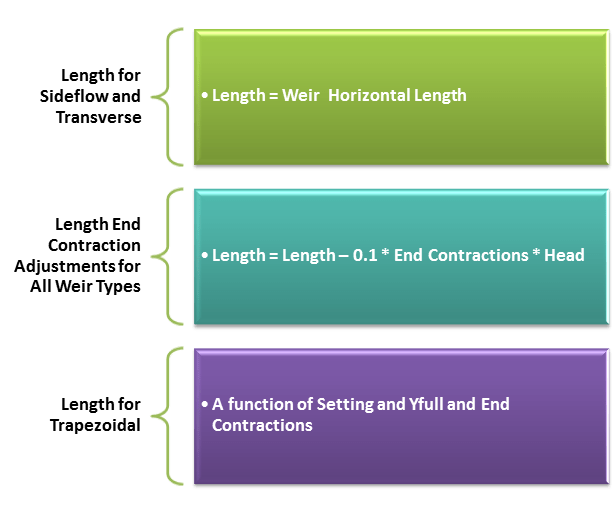#INFOSWMM

# What are the Equations for Weirs in SWMM 5, Part 2?

Subject:   What are the Equations for Weirs in SWMM 5Part 2?

There are four types of Weirs in SWMM 5:  Transverse, Sideflow, V Notch and Trapezoidal.   The trapezoidal weir is a combination of the Sideflow and V Notch Weir and the Sideflow acts like a Transverse Weir when the flow is reversed (Figure 1).  The Weirs can have zero, one or two end contractions (Figure 2) and the Weir Length is a function of the Weir Setting and Horizontal Weir Length.  A V Notch weir works as Trapezoidal Weir when the Weir RTC Setting is less than 1.0Figure 2.   Valid Number of End ContractionsFigure 3.  Weir Length Calculations